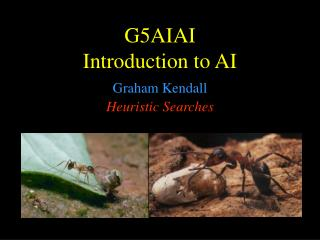DownloadDownload PresentationG5AI AI Introduction to AI

# G5AI AI Introduction to AI

Télécharger la présentation## G5AI AI Introduction to AI

- - - - - - - - - - - - - - - - - - - - - - - - - - - E N D - - - - - - - - - - - - - - - - - - - - - - - - - - -
##### Presentation Transcript

1. G5AIAIIntroduction to AI Heuristic Searches Graham Kendall

2. Heuristic Searches - Characteristics • Has some domain knowledge • Usually more efficient than blind searches • Sometimes called an informed search • Heuristic searches work by deciding which is the next best node to expand (there is no guarantee that it is the best node)

3. Heuristic Searches - Characteristics • Heuristic searches estimate the cost to the goal from its current position. It is usual to denote the heuristic evaluation function by h(n) • Compare this with something like Uniform Cost Search which chooses the lowest code node thus far ( g(n) )

4. Heuristic Searches - Implementation - 1 • Implementation is achieved by sorting the nodes based on the evaluation function; h(n)

5. Heuristic Searches - Implementation - 2 Function BEST-FIRST-SEARCH(problem, EVAL-FN) returns a solution sequence Inputs : problem, a problem Eval-Fn, an evaluation function Queueing-Fn = a function that orders nodes by EVAL-FN Return GENERAL-SEARCH(problem,Queueing-Fn)

6. Heuristic Searches - Example Hsld(n) = straight line distance between n and the goal location

7. Heuristic Searches - Greedy Search • So named as it takes the biggest “bite” it can out of the problem.That is, it seeks to minimise the estimated cost to the goal by expanding the node estimated to be closest to the goal state Function GREEDY-SEARCH(problem) returns a solution of failure Return BEST-FIRST-SEARCH(problem,h)

8. Heuristic Searches - Greedy Search • It is only concerned with short term aims • It is possible to get stuck in an infinite loop (consider being in Iasi and trying to get to Fagaras) unless you check for repeated states • It is not optimal • It is not complete Time and space complexity is O(Bm); where m is the depth of the search tree

9. Heuristic Searches - A* Algorithm • Combines the cost so far and the estimated cost to the goal.That is fn = g(n) + h(n)This gives us an estimated cost of the cheapest solution through n • It can be proved to be optimal and complete providing that the heuristic is admissible.That is the heuristic must never over estimate the cost to reach the goal • But, the number of nodes that have to be searched still grows exponentially

10. Heuristic Searches - A* Algorithm Function A*-SEARCH(problem) returns a solution of failure Return BEST-FIRST-SEARCH(problem, g + h)

11. Heuristic Searches - A* Algorithm - Example

12. Heuristic Searches - Example Problem Initial State Goal State

13. Heuristic Searches - A* Algorithm Typical solution is about twenty steps Branching factor is approximately three. Therefore a complete search would need to search 320 states. But by keeping track of repeated states we would only need to search 9! (362,880) states But even this is a lot (imagine having all these in memory) Our aim is to develop a heuristic that does not over estimate (it is admissible) so that we can use A* to find the optimal solution

14. Heuristic Searches - Possible Heuristics H1 = the number of tiles that are in the wrong position (=7) H2 = the sum of the distances of the tiles from their goal positions using the Manhattan Distance (=18) Both are admissible but which one is best?

15. Test from 100 runs with varying solution depths H2 looks better as fewer nodes are expanded. But why?

16. Effective Branching Factor • H2 has a lower branching factor and so fewer nodes are expanded • Therefore, one way to measure the quality of a heuristic is to find its average branching factor • H2 has a lower EBF and is therefore the better heuristic

17. G5AIAIIntroduction to AI End of Heuristic Searches Graham Kendall